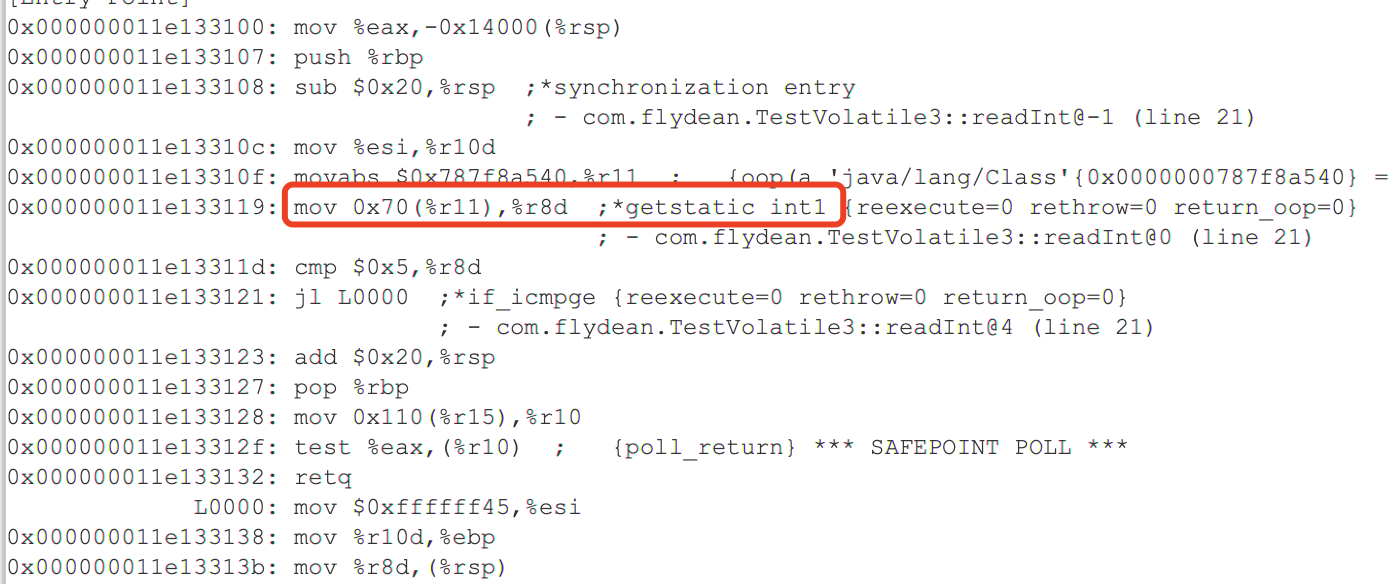# 简介

Volatile关键字对熟悉java多线程的朋友来说，应该很熟悉了。Volatile是JMM(Java Memory Model)的一个非常重要的关键词。通过是用Volatile可以实现禁止重排序和变量值线程之间可见两个主要特性。

# 重排序

``````public class TestVolatile {

private static int int1;
private static int int2;
private static int int3;
private static int int4;
private static int int5;

public static void main(String[] args) throws InterruptedException {
for (int i = 0; i < 10000; i++)
{
increase(i);
}
}

private static void increase(int i){
int1= i+1;
int2= i+2;
int3= i+3;
int4= i+4;
int5= i+5;
}
}
``````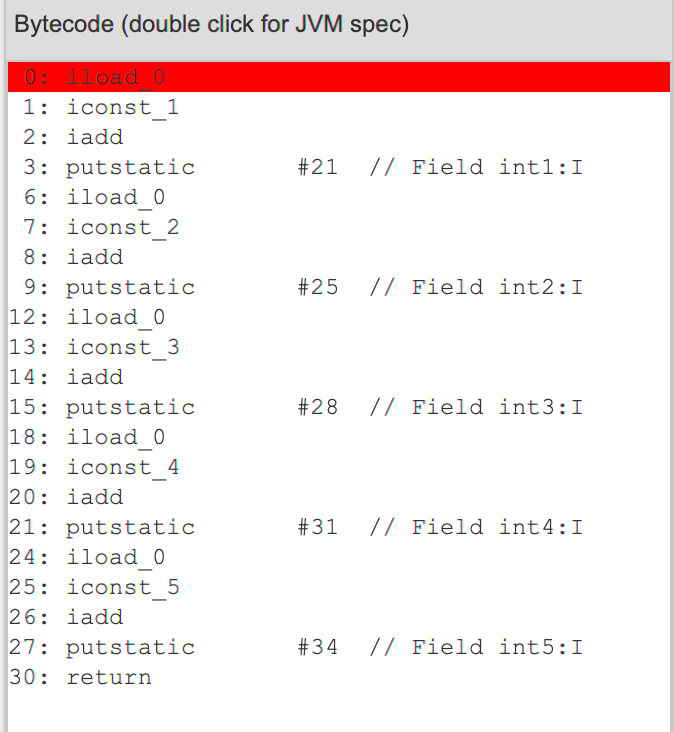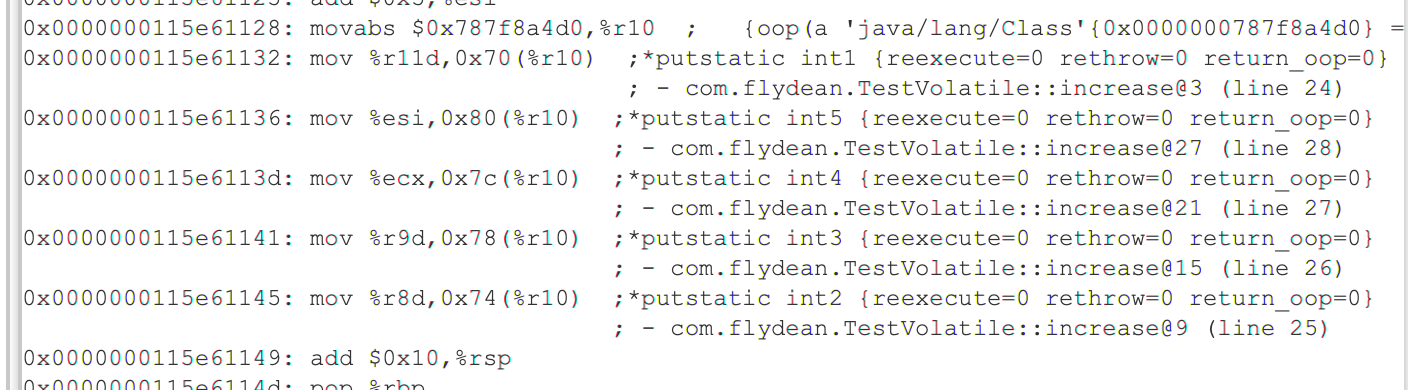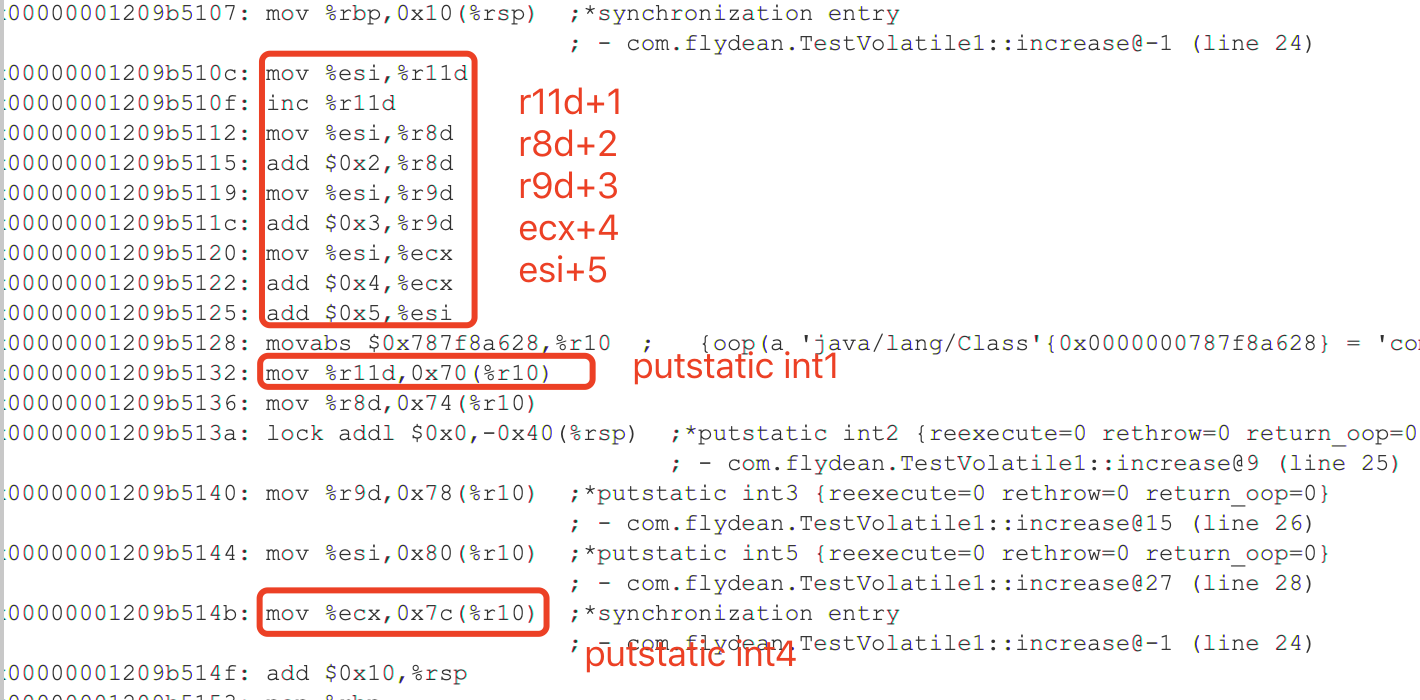5个putstatic都在，同时因为使用了volatile关键字，所以int2作为一个分界点，不会被重排序。所以int1一定在int2之前，而int3,4,5一定在int2之后。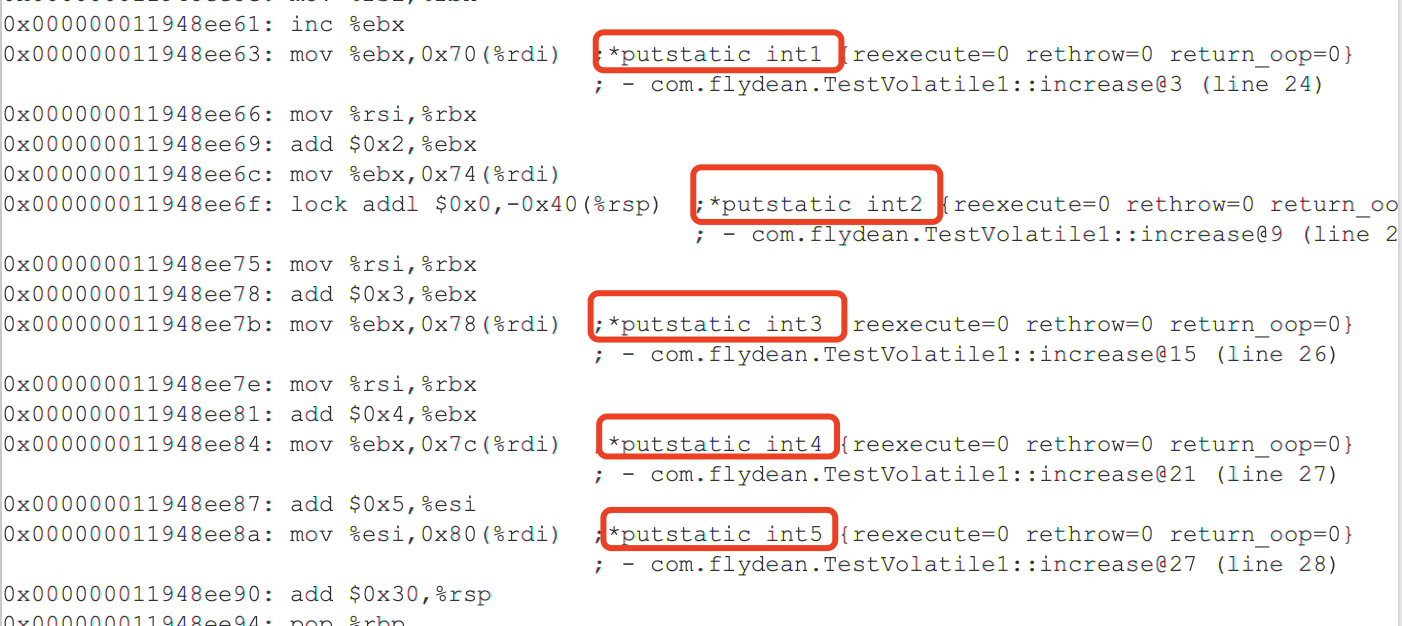# 写的内存屏障

``````lock addl \$0x0,-0x40(%rsp)  ;*putstatic int2 {reexecute=0 rethrow=0 return_oop=0}
``````

# 非lock和LazySet

``````public class TestVolatile2 {

private static int int1;
private static AtomicInteger int2=new AtomicInteger(0);
private static int int3;
private static int int4;
private static int int5;

public static void main(String[] args) throws InterruptedException {
for (int i = 0; i < 10000; i++)
{
increase(i);
}
}

private static void increase(int i){
int1= i+1;
int2.lazySet(i+2);
int3= i+3;
int4= i+4;
int5= i+5;
}
}
``````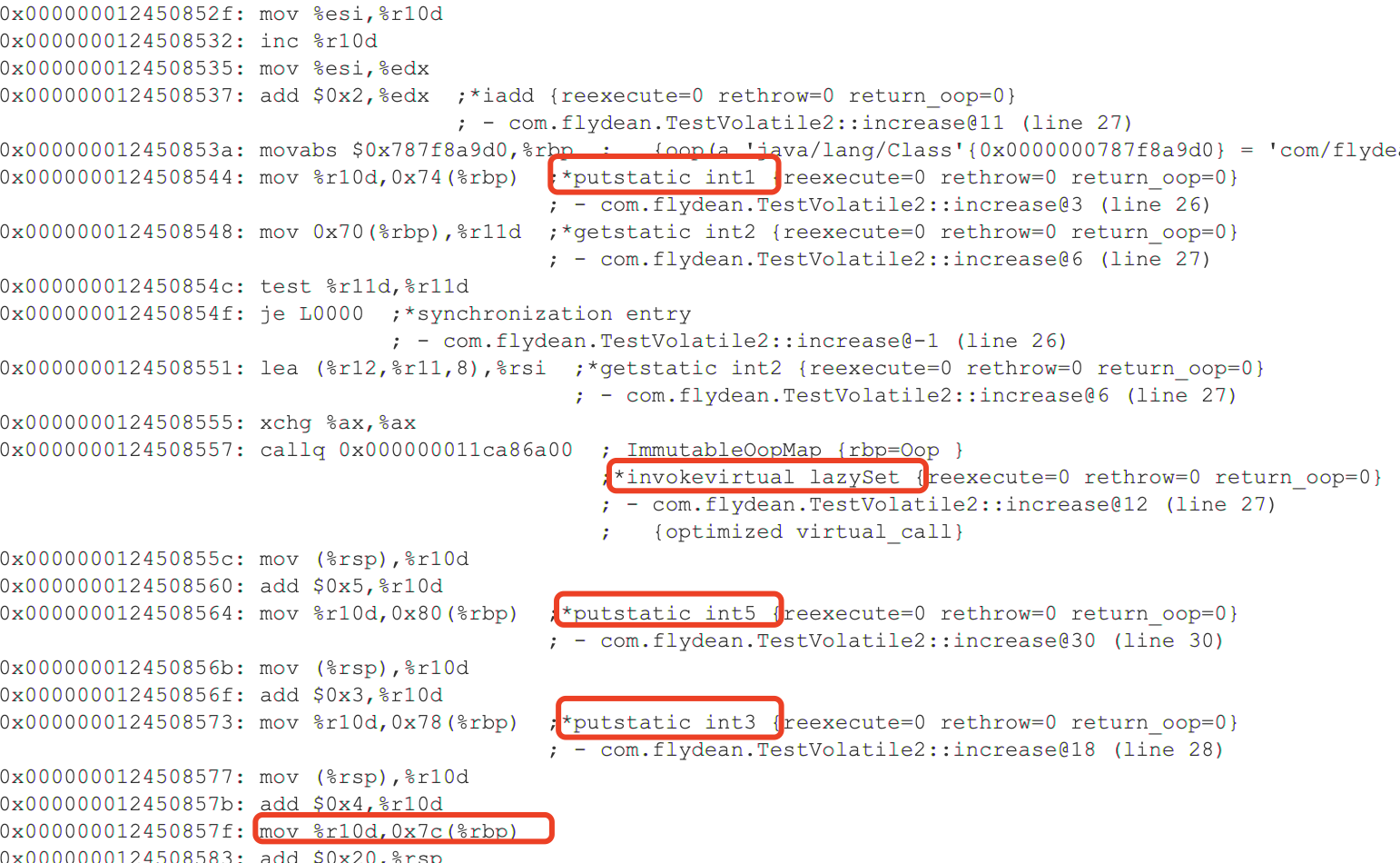# 读的性能

``````public class TestVolatile3 {

private static volatile int int1=10;
public static void main(String[] args) throws InterruptedException {
for (int i = 0; i < 10000; i++)
{
}
}

if(int1 < 5){
System.out.println(i);
}
}
}
``````